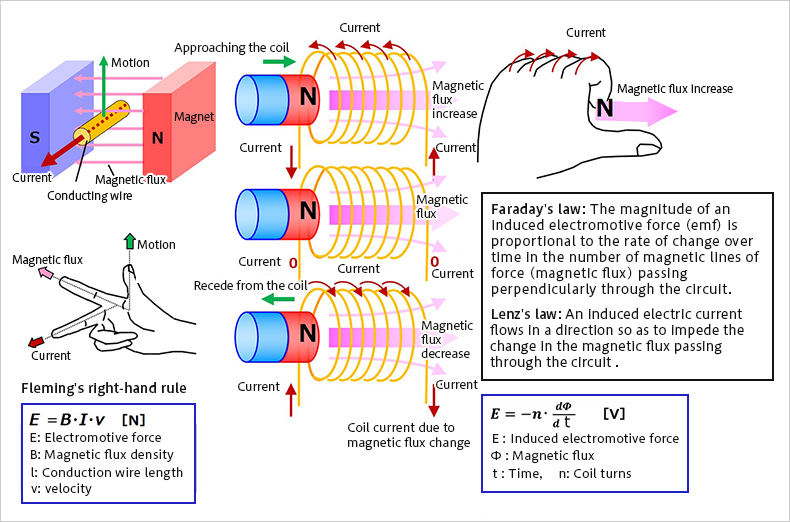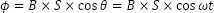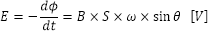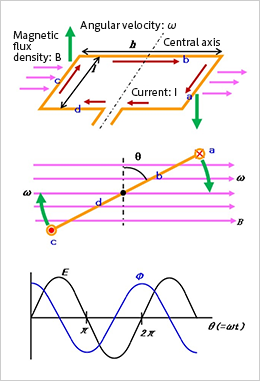# Power Generation Principle of Motors

2022.01.14

・Similarly to the rotation action, the power generation action of a motor is based on laws relating to electric currents, magnetic fields, and forces.

・Motors can convert mechanical energy (motion) into electrical energy through electromagnetic induction.

In the previous article, the principle of motor rotation was explained. Here, the principle of power generation is explained.

It was explained in the previous article that motors convert electrical energy into motive power, and rotational motion is obtained by utilizing the forces arising from the interaction between magnetic fields and electrical currents. Conversely, mechanical energy (motion) is converted into electrical energy by electromagnetic induction. That is a somewhat roundabout way of stating things, but motors have an electrical power-generating action. When we think of electric power generation, we probably imagine electric power generation equipment (also called dynamos, alternators, generators, etc.); and indeed, these have a basic construction similar in principle to that of motors. Put simply, by passing a current through the terminals of a motor, rotational motion can be obtained, and conversely, when a motor is rotated about its axis, a current flows between the terminals.

## Power Generation Action of Motors

As noted above, generation of electrical energy by a motor is due to electromagnetic induction. Below are graphic illustrations of the related laws and action for electrical energy generation.The figure on the left illustrates the flow of current according to Fleming’s right-hand rule. Motion of a conducting wire through magnetic flux induces an electromotive force in the wire, causing current to flow.

The center and right figures illustrate Faraday’s law and Lenz’s law, showing that when a magnet (magnetic flux) is made to approach or recede from a coil, current flows, and the direction of the current changes.

Based on these laws, we explain the principle of power generation.

## Power Generation Principle of Motors

Suppose that a coil of area S （＝l×h） is rotating with an angular velocity ω in a uniform magnetic field.

At this time, if the angle formed by the direction parallel to the coil plane (the yellow line in the center figure) and the line perpendicular to the direction of the magnetic flux density (the black dashed line) is θ （＝ωt）, then the magnetic flux φ passing through the coil is represented by the following equation.The induced electromotive force E occurring in the coil due to electromagnetic induction is as follows.The electromotive force is zero when the direction parallel to the coil plane is perpendicular to the magnetic flux direction, and is the absolute maximum value when the two are parallel.In this way, motors have an electric power generation action. What we intended to explain here is not that motors are used for power generation, but rather that motors have both a rotation action and a power generation action. For generating power, a generator (dynamo), which is optimized for power generation, is used.